# Samacheer Kalvi Books: Tamilnadu State Board Text Books Solutions

## Samacheer Kalvi 9th Maths Chapter 9 Probability Notes PDF Download: Tamil Nadu STD 9th Maths Chapter 9 Probability NotesSamacheer Kalvi 9th Maths Chapter 9 Probability Notes PDF Download: Tamil Nadu STD 9th Maths Chapter 9 Probability Notes

We bring to you specially curated Samacheer Kalvi 9th Maths Chapter 9 Probability Notes PDF which have been prepared by our subject experts after carefully following the trend of the exam in the last few years. The notes will not only serve for revision purposes, but also will have several cuts and easy methods to go about a difficult problem.

 Board Tamilnadu Board Study Material Notes Class Samacheer Kalvi 9th Maths Subject 9th Maths Chapter Chapter 9 Probability Format PDF Provider Samacheer Kalvi Books

## How to Download Samacheer Kalvi 9th Maths Chapter 9 Probability Notes PDFs?

2. Click on the Samacheer Kalvi 9th Maths Notes PDF.
3. Look for your preferred subject.
4. Now download the Samacheer Kalvi 9th Maths Chapter 9 Probability notes PDF.

## Tamilnadu Samacheer Kalvi 9th Maths Solutions Chapter 9 Probability Additional Questions

EXERCISE 9.1

Question 1.
An unbiased die is thrown. What is the probability of getting
(i) an even number or a multiple of 3.
(ii) a number between 3 and 6.
Solution:
(i) Probability of getting an even number 36=12
Probability of getting a multiple of 3 = 26
Probability of getting an even multiple of 3 = 16
Probability of getting an even number or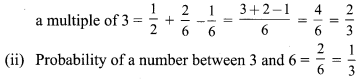Question 2.
Two unbiased coins are tossed simultaneously find the probability of getting
Solution:
S = {HH, HT, TH, TT}
(i) probability of two heads = 14
(ii) probability of one head = 12
(iii) probability of at least one head = 34
(iv) probability of at most one head = 34

Question 3.
Find the probability that a leap year selected at random will contain 53 Sundays.
Solution:
S = {Sunday Monday, Monday Tuesday, Tuesday Wednesday, Wednesday Thursday, Thursday Friday, Friday Saturday, Saturday Sunday}
n (S) = 7; n (A) = 2; P(A) = 27

Question 4.
What is the probability that a number selected from the numbers 1, 2, 3, 25 is a prime number when each of the given numbers is equally likely to be selected?
Solution:
A = {2, 3, 5, 7, 11, 13, 17, 19,23}
P(A) = 925

EXERCISE 9.2

Question 1.
Tickets numbered from 1 to 20 are mixed up together and then a ticket is drawn at random. What is the probability that the ticket has a number which is a multiple of 3 or 7?
Solution:
A = {3, 6, 9, 12, 15, 18, 7, 14}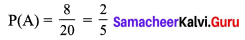Question 2.
One card is drawn from a pack of 52 cards, each of the 52 cards being equally likely to be drawn. Find the probability that the card drawn is
(i) an ace,
(ii) either red card or king.
Solution: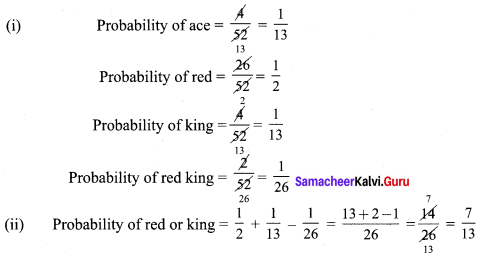Question 3.
A bag contains 3 red and 2 blue marbles. A marble is drawn at random. What is the probability of drawing a blue marble?
Solution: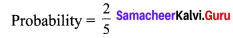Question 4.
Two dice are thrown simultaneously. Find the probability of getting
(i) an even number as the sum.
(ii) a total of at least 10
(iii) a doublet of even number.
Solution: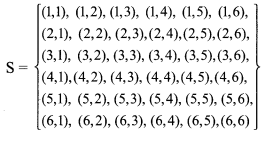n(S) = 36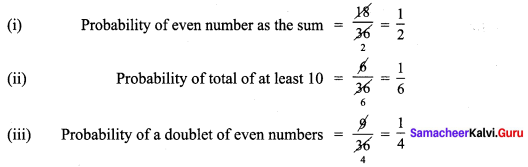Question 5.
An urn contains 10 red and 8 white balls. One ball is drawn at random. Find the probability that the ball drawn is white.
Solution:## How to Prepare using Samacheer Kalvi 9th Maths Chapter 9 Probability Notes PDF?

Students must prepare for the upcoming exams from Samacheer Kalvi 9th Maths Chapter 9 Probability Notes PDF by following certain essential steps which are provided below.

• Use Samacheer Kalvi 9th Maths Chapter 9 Probability notes by paying attention to facts and ideas.
• Pay attention to the important topics
• Refer TN Board books as well as the books recommended.
• Correctly follow the notes to reduce the number of questions being answered in the exam incorrectly
• Highlight and explain the concepts in details.

## Frequently Asked Questions on Samacheer Kalvi 9th Maths Chapter 9 Probability Notes

#### How to use Samacheer Kalvi 9th Maths Chapter 9 Probability Notes for preparation??

Read TN Board thoroughly, make separate notes for points you forget, formulae, reactions, diagrams. Highlight important points in the book itself and make use of the space provided in the margin to jot down other important points on the same topic from different sources.

#### How to make notes for Samacheer Kalvi 9th Maths Chapter 9 Probability exam?

Read from hand-made notes prepared after understanding concepts, refrain from replicating from the textbook. Use highlighters for important points. Revise from these notes regularly and formulate your own tricks, shortcuts and mnemonics, mappings etc.
Share: